Pandas DataFrame: GroupBy Examples

# Pandas DataFrame: GroupBy Examples

Last updated:

For Dataframe usage examples not related to GroupBy, see Pandas Dataframe by Example

View all examples in this post here: jupyter notebook: pandas-groupby-post

## Concatenate strings in group

This is called GROUP_CONCAT in databases such as MySQL. See below for more exmaples using the apply() function.

In the original dataframe, each row is a tag assignment.

import pandas as pd

df = pd.DataFrame({
'user_id':[1,2,1,3,3,],
'content_id':[1,1,2,2,2],
})

df.groupby("content_id")['tag'].apply(lambda tags: ','.join(tags))


After the operation, we have one row per content_id and all tags are joined with ','.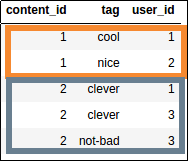Source dataframe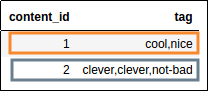All tags given to each content

## Number of unique values per group

How many unique users have tagged each movie?

import pandas as pd

df = pd.DataFrame({
'user_id':[1,2,1,3,3,],
'content_id':[1,1,2,2,2],
})

df.groupby("container_id")["user_id"].nunique().to_frame()Source dataframe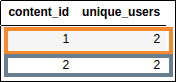How many users tagged each content?

## Sort groupby results

Turn the GroupBy object into a regular dataframe by calling .to_frame() and then reindex with reset_index(), then you call sort_values() as you would a normal DataFrame:

import pandas as pd

df = pd.DataFrame({
'value':[20.45,22.89,32.12,111.22,33.22,100.00,99.99],
'product':['table','chair','chair','mobile phone','table','mobile phone','table']
})

df1 = df.groupby('product')['value'].sum().to_frame().reset_index()

df2 = df.groupby('product')['value'].sum().to_frame().reset_index().sort_values(by='value')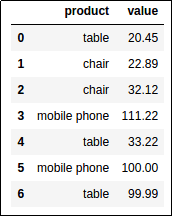Original Dataframe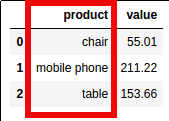Total value for each product:
df1 has the default ordering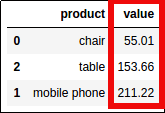Total value for each product:
df2 has been ordered by value, ascending

## Plot Groupby Count

For many more examples on how to plot data directly from Pandas see: Pandas Dataframe: Plot Examples with Matplotlib and Pyplot

If you have matplotlib installed, you can call .plot() directly on the output of methods on GroupBy objects, such as sum(), size(), etc.

import pandas as pd
import matplotlib.pyplot as plt

df = pd.DataFrame({
'value':[20.45,22.89,32.12,111.22,33.22,100.00,99.99],
'product':['table','chair','chair','mobile phone','table','mobile phone','table']
})

# use .size() to get a "count" of each group
df.groupby('product').size().plot(kind='bar')Original dataframe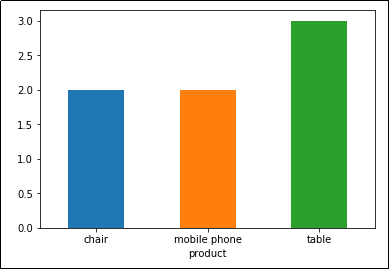Plot: Number of records by product

## Plot Groupby Sum

As above, bu using sum as the aggregator function, instead of count:

import pandas as pd
import matplotlib.pyplot as plt

df = pd.DataFrame({
'value':[20.45,22.89,32.12,111.22,33.22,100.00,99.99],
'product':['table','chair','chair','mobile phone','table','mobile phone','table']
})

df.groupby('product').sum().plot(kind='bar')Original dataframe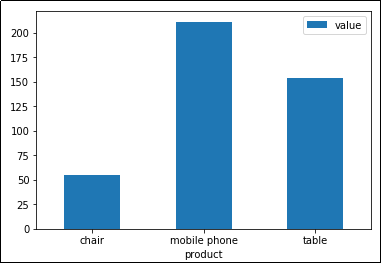Plot: Sum of column value by product.
Pandas automatically sets axes and legends too

## GroupBy Plot Mean with error bars

Add error bars (mean +/- the standard deviation1) to help people understand whether they can trust the averages or whether variance is too high:

import pandas as pd
import matplotlib.pyplot as plt

df = pd.DataFrame({
'purchase_price':[28.45, 25.89,32.12,99.99,120.00,170.00,12.22,28.22,5.00],
'product':['table','table','table','mobile phone','mobile phone','mobile phone','chair','chair','chair']
})

ax = plt.gca()

# plot the means
df.groupby('product').mean().plot(kind='bar',color='lightblue',ax=ax)

# generate a dataframe with means and standard deviations
grouped_df=df.groupby('product').agg([np.mean,np.std])

# flatten column names
grouped_df.columns = [col for col in v.columns.values]

# iterrows is usually very slow but since this is a grouped
# dataframe, there wonly be many rows
for i,(index,row) in enumerate(grouped_df.iterrows()):
name = row.name
mean = row['mean']
stddev = row['std']

# plot the vertical line for this group
ax.vlines(x=i,ymin=mean-stddev,ymax=mean+stddev)

plt.show()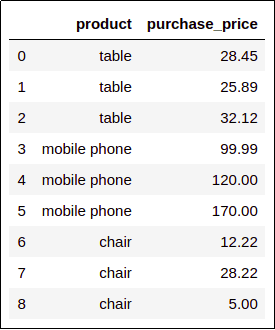Original Dataframe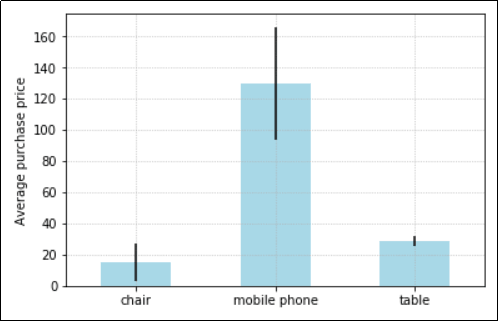The average purchase price for each product.
The black bars shows how different, on average, prices are
from the average in that group.
This helps people understand if the average can
be trusted as a good summary of the data.

## Multiple aggregations, single GroupBy pass

Say, for instance, ORDER_DATE is a timestamp column. We want to find out the total quantity QTY and the average UNIT price per day.

Note: we're not using the sample dataframe here

grouped = df.groupby(p7.ORDER_DATE.dt.day).agg({
"QTY":np.sum,
"UNIT": np.mean
})


## Flatten hierarchical indices

It's useful to execute multiple aggregations in a single pass using the DataFrameGroupBy.agg() method (see above).

But the result is a dataframe with hierarchical columns, which are not very easy to work with.

You can flatten multiple aggregations on a single columns using the following procedure:

import pandas as pd

df = pd.DataFrame({
'value':[20.45,22.89,32.12,111.22,33.22,100.00,99.99],
'product':['table','chair','chair','mobile phone','table','mobile phone','table']
})

# call
grouped_df = df.groupby('product').agg({'value':['min','max','mean']})


At this point, join together the columns, with '_' in between and the reset the index:

grouped_df.columns = ['_'.join(col) for col in grouped_df.columns.values]
grouped_df = grouped_df.reset_index()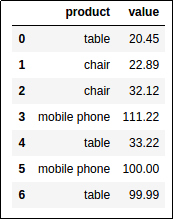Original Dataframe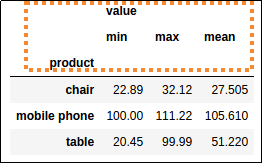The agg() method creates a hierarchical index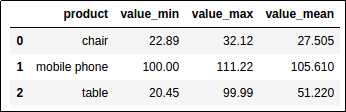Much better: Join hierarchical columns together
using '_' and then call reset_index()
for a simpler index structure

## Iterate over groups

To iterate over dataframe groups in groupby(), the object returned by the call itself can be used as an iterator:

for key,group_df in df.groupby('product'):

# key contains the name of the grouped element
# i.e. 'chair', 'mobile phone', 'table'

# group_df is a normal dataframe
# containing only the data referring to the key

print("the group for product '{}' has {} rows".format(key,len(group_df)))

# >>>
# the group for product 'chair' has 2 rows
# the group for product 'mobile phone' has 2 rows
# the group for product 'table' has 3 rows


## For group in groups

See above: Iterate over dataframe groups

## Change aggregation column name

By default, aggregation columns get the name of the column being aggregated over, in this case value

import pandas as pd

df = pd.DataFrame({
'value':[20.45,22.89,32.12,111.22,33.22,100.00,99.99],
'product':['table','chair','chair','mobile phone','table','mobile phone','table']
})

grouped_df = df.groupby('product')['value'].sum().reset_index(name='value_sum')Original Dataframe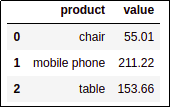By default, aggregation columns get the name of the
column being aggregated over, in this case value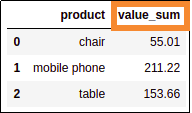Give it a more intuitive name using reset_index(name='new name')

## Get group by key

After calling groupby(), you can access each group dataframe individually using get_group().

import pandas as pd

df = pd.DataFrame({
'value':[20.45,22.89,32.12,111.22,33.22,100.00,99.99],
'product':['table','chair','chair','mobile phone','table','mobile phone','table']
})

# grouped_df is a DataFrameGroupBy containing each individual group as a dataframe
grouped_df = df.groupby('product')

# you get can a dataframe containing the values for a single group
# using .get_group('group_key')
grouped_df.get_group('chair')Original dataframe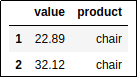Results of .get_group('chair'): only
results in 'chair' group are returned

## List values in group

See below for more examples using the apply() function.

Use apply with a custom function.

For example, get a list of the prices for each product:

import pandas as pd

df = pd.DataFrame({
'value':[20.45,22.89,32.12,111.22,33.22,100.00,99.99],
'product':['table','chair','chair','mobile phone','table','mobile phone','table']
})

# note that the apply function here takes a series made up of the values
# for each group. We then call the .tolist() method on the series to make
# it into a list
df.groupby('product')['value'].apply(lambda group_series: group_series.tolist()).reset_index()Original dataframe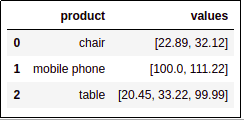Group values for each group into a list

## Custom aggregation

Use apply(func) where func is a function that takes a Series representing a single group and reduces that Series to a single value.

Example: get count of even values in each group

import pandas as pd

df = pd.DataFrame({
'value':[20,22,32,111,33,100,99],
'product':['table','chair','chair','mobile phone','table','mobile phone','table']
})

# you can define a function like this or use a lambda function
def count_even_numbers(series):
return len([elem for elem in series if elem % 2 == 0 ])

df.groupby('product')['value'].apply(count_even_numbers).reset_index(name='num_even_numbers')Original dataframe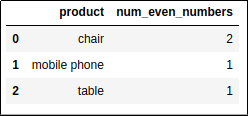How many even-numbered values in each group?

## Sample rows after groupby

This is also called stratified sampling.

The easiest way to do this is df.groupby(<colname>).apply:

import pandas as pd

df = pd.DataFrame({
'price':[20,22,32,111,33,100,99],
'product':['table','chair','chair','table','table','chair','table']
})

# you could just as easily group by multiple columns here
df.groupby("product").apply(
lambda group_df: group_df.sample(2) # any dataframe function could be used here
).reset_index(drop=True) # fixes the index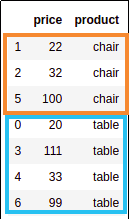Source dataframe with chairs and tables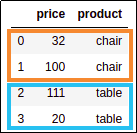Sampled dataframe, with 2 samples
per group.